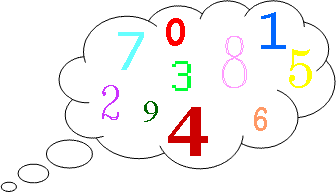Here is an alternative version of the “Think of a Number” trick.

Think of two whole numbers under 10.
Take one of them and add 1.
Multiply by 5.
Subtract 1.
Double again.
Subtract 8.

I can work out both your numbers very quickly. How?

Choose some different pairs of numbers and repeat the process.
Can you find a good explanation of how the trick works?